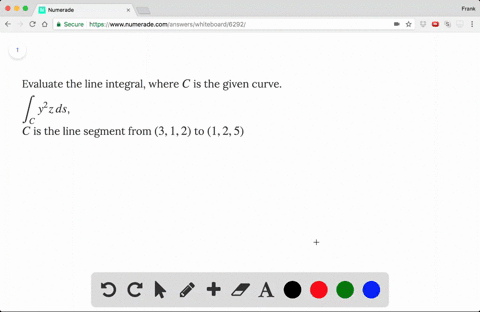🎉 The Study-to-Win Winning Ticket number has been announced! Go to your Tickets dashboard to see if you won! 🎉View Winning Ticket01:50
FL
Problem 9

# Evaluate the line integral, where $C$ is the given curve.$\displaystyle \int_C x^2y \, ds$, $C: x = \cos t$, $y = \sin t$, $z = t$, $0 \leqslant t \leqslant \pi/2$

## Discussion

You must be signed in to discuss.

## Video Transcript

we're through this light the crow. So first way Haven't the DSR first funeral for this? Yes. So d x d t is negative side he Teo wide Tichy is co sign T please is one so yes should be squared off Science qwerty plus coz I scurti plus y squared tichy on the square root off to TT So we just do the integral he from zero to pie over too X square is coz I scurti side yes square root of to dt or use a U substitution. So cause I tease we said you host co society So this one No bu square and science the negative sign titties to you We have minor square root of to you squared you when she goes from zero to pie over too co signed he goes from from one to zero So it's a semi zero to one square root of to you squared you I got you cube over three employing one So you just got square root of two times one over Siri